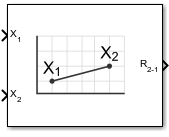Main Content

# Calculate Range

Calculate range between two vehicles given their respective positions

• Library:
• Aerospace Blockset / GNC / Guidance

•## Description

The Calculate Range block computes the range between two vehicles. The equation used for the range calculation is

`$Range=\sqrt{{\left({x}_{1}-{x}_{2}\right)}^{2}+{\left({y}_{1}-{y}_{2}\right)}^{2}+{\left({z}_{1}-{z}_{2}\right)}^{2}}$`

## Ports

### Input

expand all

Contains the (x, y, and z) position of vehicle 1, specified as a three-element vector. These values of the double data type.

Data Types: `double`

The (x, y, and z) position of vehicle 2, specified as a three-element vector. These values are of the double data type.

Data Types: `double`

### Output

expand all

Range from vehicle 2 and vehicle 1, returned as a scalar of double data type. The calculated range is the magnitude of the distance, but not the direction. It is always positive or zero.

Data Types: `double`

## See Also

Introduced before R2006a

## SupportGet trial now